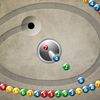# Math man

Prepare to indulge in the remake of the phenomenal arcade classic and journey through the amazing arithmetical world! Your goal in this game is to control the Math Man to consume all the ghosts in the mazes after solving the equations. When the game opens, the ghosts will start to hunt the Math Man. Each of the ghosts is marked by a number which represents the answer of an equation. Use the four arrow keys on your keyboard to control the Math Man, and move him to the location of the special food labeled with a question mark. Then an equation will appear at the bottom of the screen, and you need to find out the answer. You may also revisit the special food to receive another equation. When you have obtained the answer, the Math Man can eat the ghost with the number of the answer. Then you need to visit the special food again to receive another question. Continue the process until all ghosts are consumed, and you can proceed to the next level. The Math Man will start the game with 3 live

## Related software (5)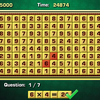## Arithmetic Game

Complete the equations in the interesting arithmetic test!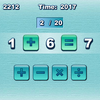## Quick Math

How quickly can you complete the equations with arithmetic symbols?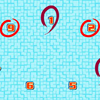## Make 15

Pick 3 numbers that add up to 15.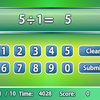## Quick Calculate